# 机器学习、深度学习必备数学基础之常用的距离

### 1. 曼哈顿距离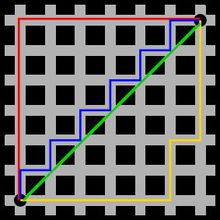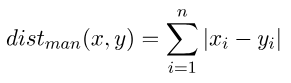import numpy as np

vector1 = np.mat([[1, -2, 3], [1, 2, 3]])
vector2 = np.mat([[4, 5, -6], [4, 5, 6]])
print(np.sum(np.abs(vector1 - vector2)))      # 28

### 2. 欧氏距离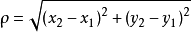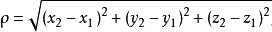n 维空间：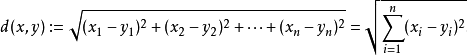import numpy as np

vector1 = np.mat([[1, -2, 3], [1, 2, 3]])
vector2 = np.mat([[4, 5, -6], [4, 5, 6]])
print(np.sqrt((vector1 - vector2) * (vector1 - vector2).T))
# [[11.78982612  1.73205081]
#  [ 1.73205081  5.19615242]]

### 3. 切比雪夫距离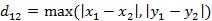n 维向量：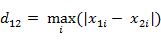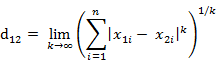from numpy import *

vector1 = np.mat([[1, -2, 3], [1, 2, 3]])
vector2 = np.mat([[4, 5, -6], [4, 5, 6]])
print(np.max(np.abs(vector1 - vector2)))    # 9

### 4. 闵可夫斯基距离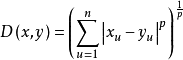（1）闵氏距离与特征参数的量纲有关，有不同量纲的特征参数的闵氏距离常常是无意义的。

（2）闵氏距离没有考虑特征参数间的相关性，没有考虑各个特征参数的分布（期望，方差等)可能是不同的。

### 5. 汉明距离

1011101 与 1001001 之间的汉明距离是 2。

2143896 与 2233796 之间的汉明距离是 3。

"toned" 与 "roses" 之间的汉明距离是 3。

from numpy import *

vector1 = mat([1, 1, 0, 1, 0, 1, 0, 0, 1, 1])
vector2 = mat([0, 1, 1, 0, 0, 0, 1, 1, 1, 0])
nonzero_str = nonzero(vector1 - vector2)
print(nonzero_str)  # (array([0, 0, 0, 0, 0, 0, 0], dtype=int64), array([0, 2, 3, 5, 6, 7, 9], dtype=int64))
# nonzero_str 为对应位置的不同元素所在的索引
print(shape(nonzero_str))  # 7

### 6. 夹角余弦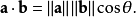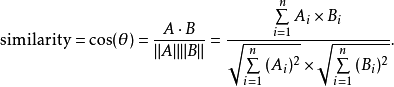from numpy import *
vector1 = mat([1,2,3])
vector2 = mat([4,5,6])
print dot(vector1,vector2)/(linalg.norm(vector1)*linalg.norm(vector2))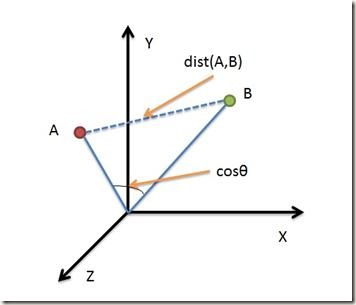• 欧氏距离能够体现个体数值特征的绝对差异，所以更多的用于需要从维度的数值大小中体现差异的分析，如使用用户行为指标分析用户价值的相似度或差异。
• 余弦距离更多的是从方向上区分差异，而对绝对的数值不敏感，更多的用于使用用户对内容评分来区分兴趣的相似度和差异，同时修正了用户间可能存在的度量标准不统一的问题（因为余弦距离对绝对数值不敏感）。

### 7. 杰卡德相似系数与杰卡德距离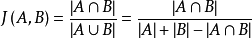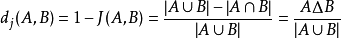1. 比较文本相似度，用于文本查重与去重；

2. 计算对象间距离，用于数据聚类等。

3. 推荐系统。与传统相似性度量方法相比，杰卡德方法在基于物品的协同过滤系统中建立评价分析方法，完善了余弦相似性只考虑用户评分而忽略了其他信息量的弊端，特别适合于应用到稀疏度过高的数据中。

import numpy as np
import scipy.spatial.distance as dist

matv = np.array([[1, 1, 0, 1, 0, 1, 0, 0, 1], [0, 1, 1, 0, 0, 0, 1, 1, 1]])
print(matv)
# [[1 1 0 1 0 1 0 0 1]
#  [0 1 1 0 0 0 1 1 1]]

ds = dist.pdist(matv, 'jaccard')
print(ds)   # [0.75]

### 8. 马氏距离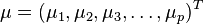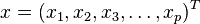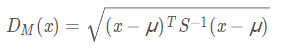1. 马氏距离不受量纲的影响，两点之间的马氏距离与原始数据的测量单位无关。

2. 由标准化数据和中心化数据（即原始数据与均值之差）计算出的二点之间的马氏距离相同。

3. 马氏距离还可以排除变量之间的相关性的干扰。

1. 夸大了变化微小的变量的作用。

2. 马氏距离的计算是不稳定的，主要取决于协方差矩阵（相同的样本在不同的总体样本集中计算得出的结果通常是不同的），这也是马氏距离与欧式距离的最大差异之处。

import numpy as np

## 根据公式求解

# 由于马氏距离要求样本数要大于维数，否则无法求协方差矩阵。（此前提在生产中一般都满足）
x = np.random.random(5)
y = np.random.random(5)

# 构建二行五列的数组
X = np.vstack([x, y])

# 0. 对数组转置，转置后的结果为十行五列的数组，表示5个样本，每个样本2个维度
XT = X.T

# 1. 构建两个维度之间协方差矩阵
S = np.cov(X)

# 2. 协方差矩阵的逆矩阵
ST = np.linalg.inv(S)

# 3. 马氏距离计算两个样本之间的距离，此处共有5个样本，两两组合，共有10个距离（排列组合五个中取两个，C 5 2）
# 取当前样本数
samples_num = XT.shape
result_dis = []
# 遍历每个样本，计算样本向量到协方差矩阵的距离
for i in range(0, samples_num):
for j in range(i + 1, samples_num):
# 对应公式中的 x-u
vector_difference = XT[i] - XT[j]
# 计算点积后开方，获取距离
dis = np.sqrt(np.dot(np.dot(vector_difference, ST), vector_difference.T))
result_dis.append(dis)

print(result_dis)
# [0.9005405956748153, 1.9326547704124346, 1.449159387238744, 2.5878730470795053, 2.5813933696678806, 0.9425573033749342, 2.326197750393925, 2.3802959458987134, 2.4656065983430806, 1.3958852659218475]

### 参考链接

https://www.cnblogs.com/denny402/p/7027954.html

https://blog.csdn.net/bluesliuf/article/details/88862918

05-26407

#### 深度学习理论方法-余弦相似度计算实战与欧几里得距离概述

01-08151

#### 机器学习、深度学习必备数学基础之线性代数篇09-2840万+

#### BAT机器学习面试1000题系列（第1~305题）

12-20255

#### 深度学习工作笔记002---曼哈顿距离_欧氏距离

02-1999

#### 基于keras的深度学习基本概念讲解——深度学习之从小白到入门

04-293238

#### Deep Learning（深度学习）学习笔记整理系列之常用模型（四、五、六、七）

03-061万+

#### 深度学习中常用损失函数

07-133610

#### 深度学习常用中英文对照表

03-302689

#### 深度学习常用损失函数

04-271675

#### caffe+报错︱深度学习参数调优杂记+caffe训练时的问题+dropout/batch Normalization©️2020 CSDN 皮肤主题: 精致技术 设计师: CSDN官方博客点击重新获取扫码支付1.余额是钱包充值的虚拟货币，按照1:1的比例进行支付金额的抵扣。
2.余额无法直接购买下载，可以购买VIP、C币套餐、付费专栏及课程。余额充值